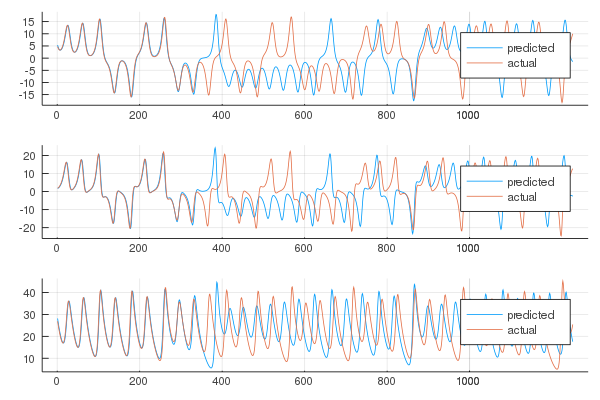# Basics

The following is a basic example that will introduce the reader to the function and structs of the ReservoirComputing library.

The goal for this example is to predict the Lorenz system, so first we need to obtain the data.

using ParameterizedFunctions, OrdinaryDiffEq

#lorenz system parameters
u0 = [1.0,0.0,0.0]
tspan = (0.0,1000.0)
p = [10.0,28.0,8/3]

#define lorenz system
function lorenz(du,u,p,t)
du = p*(u-u)
du = u*(p-u) - u
du = u*u - p*u
end

#solve system
prob = ODEProblem(lorenz, u0, tspan, p)
sol = solve(prob, ABM54(), dt=0.02)
v = sol.u
data = Matrix(hcat(v...))

Now that we have the data, we can create two datasets, one for the training and another to test the results obtained:

shift = 300
train_len = 5000
predict_len = 1250

#get data
train = data[:, shift:shift+train_len-1]
test = data[:, shift+train_len:shift+train_len+predict_len-1]

It is always a good idea to add a shift in order to wash out any initial transient. Having the data, we can proceed to creating the ESN for the prediction:

using ReservoirComputing
#model parameters
approx_res_size = 300 #size of the reservoir
activation = tanh #neuron activation function
degree = 6 #degree of connectivity of the reservoir
sigma = 0.1 # input weight scaling
beta = 0.0 #ridge
alpha = 1.0 #leaky coefficient
nla_type = NLAT2() #non linear algorithm for the states
extended_states = false # if true extends the states with the input

#create echo state network
esn = ESN(approx_res_size,
train,
degree,
activation = activation,
sigma = sigma,
alpha = alpha,
nla_type = nla_type,
extended_states = extended_states)

Since this is an introductory example, we wanted to show all possible parameters, even though the same values that we defined are the default ones of the ESN constructor. For the training and the prediction we just need the following two lines:

W_out = ESNtrain(esn, beta)
output = ESNpredict(esn, predict_len, W_out) #applies standard ridge regression for the training

Now, if we want to check the results, we can plot the output and the test dataset:

using Plots
plot(transpose(output),layout=(3,1), label="predicted")
plot!(transpose(test),layout=(3,1), label="actual")